PHY294H - Lecture 45

Multiple slit grating

Light which travels through a set of N parallel slit, with slits which are very narrow, leads to the intensity pattern,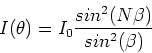(1)

where I0 is the intensity due to one slit and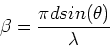(2)

If we take into account the width of the slits, taking a to be the slit width, we get,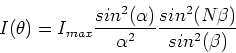(3)

where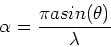(4)

x-ray diffraction, Von Laue (1912)

Crystal lattices have regular spacings, so if light is reflected from them, the scattered light looks like the light being emmitted from a set of point sources. There are intensity maxima at angles which are given by (for a geometry where the incoming light is at angle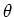to a crystal plane and we measure the outgoing light at the same angle,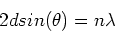(5)

where d is the separation between a set of crystal planes. This is called Bragg reflection. The factor of two occurs because in reflection, the extra pathlength is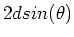. For each set of crystal planes there is a series of reflections. There is then a large set of Bragg peaks'' when the crystal is of high quality. By analysing the set of Bragg peaks it is possible to determine the spacings between all of the planes in a crystal and hence to deduce the crystal structure. This can be simple if the crystal structure is simple, but it is complex if the crystal structure is complex (e.g. DNA).

Diffraction limits on resolution

To understand diffraction limits on resolution of microscopes and telescopes, we first need to understand the diffraction pattern due to a circular hole of diameter D. The diffraction pattern due to such a hole is a set of concentric light and dark rings. The first maximum is at zero angle (of course) and the first minimum is at an angle of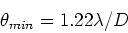(6)

For a single slit the first minimum is at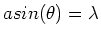which implies that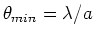. Two sources are just resolved when their angular separation is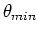. If their angular separation is smaller than, then the images of the two sources merge together into one unresolved image. In many cases the factor of 1.22 in the equation above is dropped from the analysis.

Resolution of the compound microscope

The object is placed at the focal length of the objective lens. The separation of features that can be resolved on the microscope slide is then(using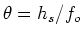),(7)

where D is the diameter of the objective lens.

Resolution of the human eye A similar calculation can be applied to the human eye, where we take D = 2.5mm and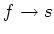.

Resolution of telescopes

The angular resulution of telescopes is simply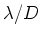, where D is the diameter of the primary lens or mirror. Earth bound telescopes have less resolution than this due to atmospheric turbulence. The Hubble space telescope has resolution which is close to the diffraction limit. Spy satellites have the same problem as earth bound telescopes and the best resolution they have, in observing objects on earth, is about 1m.

For both telescopes and microscopes the first lens or mirror that the incoming light is incident up is the most important for the diffraction limit on resolution. If the sources are not resolved by the first element in the optical system, then the other optical elements cannot help. In addition the angle of the intermediate image is amplified, making the diffraction effects of the later elements less important.